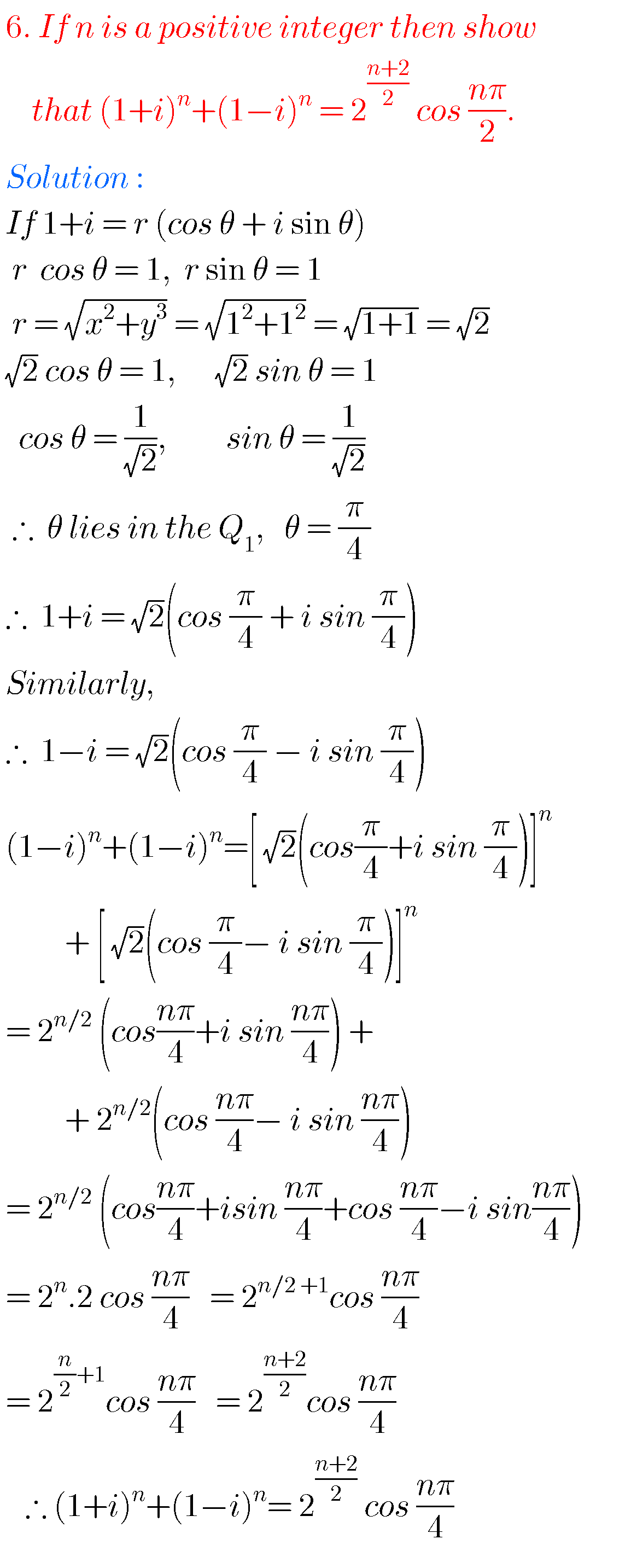## NCERT solutuons for class 7th maths chapter 2/fractions and decimals solutions

NCERT SOLUTIONS FOR CLASS 7TH MATHS CHAPTER 2, FRACTIONS AND DECIMALS You can see the solutions of some chapters for 7 th class maths. Ncert solutions for class maths chapter 1 integers Chapter 4 simple equations solutions 3. Rational numbers solutions Ncert solutions for class 6 th maths some chapters. Real numbers solutions class 10 …## Hyperbolic functions,Intermediate first year 1 A chapter 9 problems with solutions,trigonometry hyperbolic functions junior inter

HYPERBOLIC FUNCTIONS, INTERMEDIATE FIRST YEAR 1 A PROBLEMS WITH SOLUTIONS Intermediate first year maths 1A and 1B some chapters. The solutions are very simple to understand. Inter 1st year 1A : Functions, mathematical induction, functions, addition of vectors, trigonometric ratios upto transformations, trigonometric equations, hyperbolic functions, inverse trigonometric functions and properties of triangles. Inter 1st year …## MATHEMATICAL INDUCTION, Intermediate 1st year problems with solutions,solutions for mathematical induction inter first year 1A

MATHEMATICAL INDUCTION, INTERMEDIATE FIRST YEAR PROBLEMS WITH SOLUTIONS Mathematics intermediate first year 1A and 1B solutions for some problems. These solutions are very simple to understand.  Junior inter 1A : Functions, mathematical induction, functions, addition of vectors, trigonometric ratios upto transformations, trigonometric equations, hyperbolic functions, inverse trigonometric functions and properties of triangles. Junior inter 1B : …## Trigonometric ratios upto transformations,intermediate first year 1A problems with solutions

TRIGONOMETRIC RATIOS UPTO TRANSFORMATIONS, 2 Mathematics Intermediate 1st year mathematics 1A and 1B solutions for some problems. These solutions are very easy to understand. Inter first year 1A : Functions, mathematical induction, addition of vectors, trigonometric ratios upto transformations, trigonometric equations, hyperbolic functions, inverse trigonometric functions and properties of triangles. Inter first year 1B : Locus, transformation …## Properties of triangles, Intermediate mathematics first year problems with solutions,solutions of properties of triangles, junior inter trigonometry

PROPERTIES OF TRIANGLES, INTERMEDIATE MATHEMATICS FIRST YEAR PROBLEMS WITH SOLUTIONS Intermediate mathematics first year 1A and 1B some chapters. The solutions are very easy to understand. Inter first year 1A : Functions, mathematical induction, functions, addition of vectors, trigonometric ratios upto transformations, trigonometric equations, hyperbolic functions, inverse trigonometric functions and properties of triangles. Inter first year …## Trigonometric equations,intermediate mathematics first year 1 A chapter 7 problems with solutions,junior inter trigonometry

TRIGONOMETRIC EQUATIONS, INTERMEDIATE FIRST YEAR 1 A CHAPTER 7 PROBLEMS WITH SOLUTIONS Mathematics intermediate 1A and 1B solutions for some problems. These solutions are very simple to understand. Inter first year 1A :  Solutions for functions, mathematical induction, addition of vectors, trigonometric ratios upto transformations, trigonometric equations, hyperbolic functions, inverse trigonometric functions and properties of …

## Functions,intermediate,Solutions of functions intermediate mathematics 1A/functions inter first year problems with solutions/ junior inter mathematics algebra/solutions of functions Intermediate first year

SOLUTIONS OF FUNCTIONS INTERMEDIATE MATHEMATICS 1 A Intermediate maths first year 1A and 1B some chapters solutions. The solutions are very simple to understand. First year 1A :  Functions, mathematical induction, functions, addition of vectors, trigonometric ratios upto transformations, trigonometric equations, hyperbolic functions, inverse trigonometric functions and properties of triangles. First year 1B :  Locus, transformation …## Trigonometric Ratios upto transformations intermediate mathematics first year 1A problems with solutions/trigonometry

INTERMEDIATE MATHEMATICS PROBLEMS WITH SOLUTIONS Solutions for inter mathematics first year 1A and 1B some chapters. The solutions are very simple to understand. Junior inter 1A : Functions, mathematical induction, functions, addition of vectors, trigonometric ratios upto transformations, trigonometric equations, hyperbolic functions, inverse trigonometric functions and properties of triangles solutions. Junior inter 1B : Solutions for …## Inter maths solutions for Quadratic expressions second year,intermediate maths 2a(IIA) chapter 3 exercise 3(a) text book solutions

Inter maths solutions for Quadratic expressions second year Intermediate mathematics second year solutions for quadratic expressions for some problems. These solutions are very easy to understand. These are helpful for examination purpose.  You can see the solutions for maths IIA inter  1. Complex numbers 2. De Mouvre’s theorem 3. Quadratic expressions exercise 3(a) Exercise 3(b) Exercise 3(c) 4. Theory …## Inter maths solution for De Moivre’s theorem,intermediate 2nd year mathematics IIA(2a) chapter 2 solutions

Inter maths solution for De Moivre’s theorem Mathematics intermediate second year maths IIA solutions for some problems. These are very easy to understand. Inter maths solutions for first year 1a and 1b for examination purpose. Inter maths 2a solutions for complex numbers and de moivre’s theorem. Inter first year 1a and 1b solutions are also …